# GROUP BY Clause in Oracle

## GROUP BY Clause in Oracle with Examples

In this article, I am going to discuss the GROUP BY Clause in Oracle with Examples. Please read our previous article where we discussed the ORDER BY Clause in Oracle with Examples. At the end of this article, you will understand the following pointers.

1. What is Group by Clause in Oracle?
2. GROUP BY Clause with the COUNT Function in Oracle
3. GROUP BY Clause with SUM Function
4. GROUP BY Clause with MAX and MIN Function
5. GROUP By Clause with Multiple Columns in Oracle
6. GROUP BY Clause with AVG Function in Oracle
##### What is Group by Clause in Oracle?

The GROUP BY clause in Oracle is used to group the data together. It aggregates many rows into one. The FROM and WHERE clause creates an intermediate tabular result set and the GROUP BY clause systematically groups the data. The GROUP BY clause can group the result set by one or more columns. That means the Group by Clause divides similar types of records or data as a group and then returns. If we use group by clause in the query then we should use grouping/aggregate functions such as COUNT(), SUM(), MAX(), MIN(), AVG() functions.

When we implement group by clause, first the data of the table will be divided into separate groups as per the column and later aggregate function will execute on each group data to get the result. That means the first GROUP BY clause is used to divide similar types of data as a group and then the aggregate function is applied to each group to get the required results.

##### Syntax to use GROUP BY Clause in Oracle

Following is the syntax to use Group by Clause in Oracle.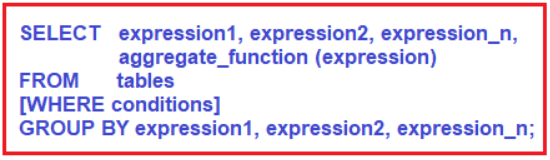##### Parameters or Arguments used in Group by Clause in Oracle:
1. expression1, expression2, expression_n: The expressions that are not encapsulated within an aggregate function must be included in the GROUP BY clause.
2. aggregate_function: The aggregate function can be SUM, COUNT, MIN, MAX, or AVG functions that we should use while we are using the Group by Clause in Oracle.
3. Tables: Tables are nothing but the name of the table or tables from which we want to retrieve the data.
4. WHERE conditions: It is optional. If you want to retrieve the data based on some conditions then you need to specify such conditions using the WHERE Clause.

Note: The GROUP BY clause in Oracle should be placed after the FROM and WHERE clauses. Again, there must be at least one column or one expression after the GROUP BY keyword, that you want to use as criteria to group the records or rows.

##### Example to understand GROUP BY Clause in Oracle:

We are going to use the following Employee table to understand the GROUP BY Clause in Oracle with Examples.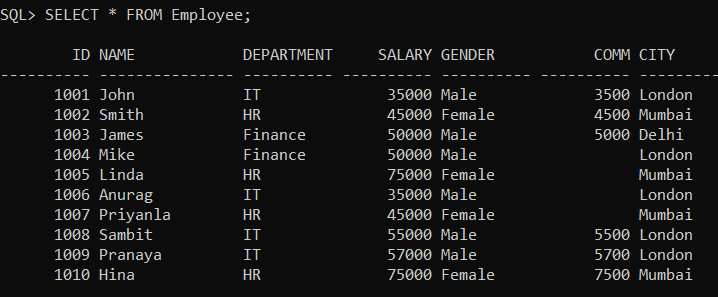Please execute the below SQL query to drop the existing Employee table if any and create a new Employee table with the required data.

```DROP Table Employee;

CREATE TABLE Employee (
Id INT PRIMARY KEY,
Name VARCHAR(15),
Department VARCHAR(10),
Salary NUMBER(8, 2),
Gender VARCHAR(10),
Comm INT,
City VARCHAR(10)
);

INSERT INTO Employee (Id, Name, Department, Salary, Gender, Comm, City) VALUES (1001, 'John', 'IT', 35000, 'Male', 3500, 'London');
INSERT INTO Employee (Id, Name, Department, Salary, Gender, Comm, City) VALUES (1002, 'Smith', 'HR', 45000, 'Female', 4500, 'Mumbai');
INSERT INTO Employee (Id, Name, Department, Salary, Gender, Comm, City) VALUES (1003, 'James', 'Finance', 50000, 'Male', 5000, 'Delhi');
INSERT INTO Employee (Id, Name, Department, Salary, Gender, Comm, City) VALUES (1004, 'Mike', 'Finance', 50000, 'Male', NULL, 'London');
INSERT INTO Employee (Id, Name, Department, Salary, Gender, Comm, City) VALUES (1005, 'Linda', 'HR', 75000, 'Female', NULL, 'Mumbai');
INSERT INTO Employee (Id, Name, Department, Salary, Gender, Comm, City) VALUES (1006, 'Anurag', 'IT', 35000, 'Male', NULL, 'London');
INSERT INTO Employee (Id, Name, Department, Salary, Gender, Comm, City) VALUES (1007, 'Priyanla', 'HR', 45000, 'Female', NULL, 'Mumbai');
INSERT INTO Employee (Id, Name, Department, Salary, Gender, Comm, City) VALUES (1008, 'Sambit', 'IT', 55000, 'Male', 5500, 'London');
INSERT INTO Employee (Id, Name, Department, Salary, Gender, Comm, City) VALUES (1009, 'Pranaya', 'IT', 57000, 'Male', 5700, 'London');
INSERT INTO Employee (Id, Name, Department, Salary, Gender, Comm, City) VALUES (1010, 'Hina', 'HR', 75000, 'Female', 7500, 'Mumbai');
```
##### GROUP BY Clause with the COUNT Function in Oracle

Now let us see some examples of using Group by Clause with COUNT aggregate function in Oracle. The COUNT aggregate function in Oracle is going to return the count of the total number of records.

###### Example: Count the total number of employees in the Employee table

SELECT COUNT(*) AS TotalEmployee FROM Employee;
Output: 10

As the employee table contains a total of 10 records, so the count function returns 10. As you can see, here we are not using any group by function.

###### Example: Count the total number of employees in each department from the Employee table

So, here first we need to group the employees by department, and then we need to execute the count aggregate function on each group. The following SQL Query exactly does the same.

SELECT Department, COUNT(*) AS TotalEmployee FROM Employee GROUP BY Department;

Once you execute the above query, you will get the following output which is showing the department name along with the number of employees of belongs to that department.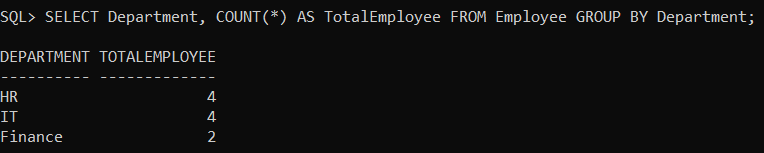##### GROUP BY Clause with SUM Function in Oracle

Now let us see an example of using Group by Clause with SUM aggregate function in Oracle. The SUM aggregate function in Oracle is going to sum the value on the column on which it is operated.

###### Example: Find the total salary given to all employees

SELECT SUM(Salary) as TotalSalary FROM Employee;
Output: 522000

###### Example: Count the total salary given to each department in the employee table

Here first we need to group the employees by department and then we need to execute the SUM aggregate function on each group. And to SUM function we need to pass the Salary column. The following SQL Query exactly does the same.

SELECT Department, SUM(Salary) as TotalSalary FROM Employee GROUP BY Department;

When you execute the above query, you will get the following output which is showing the department name along with the total salary given to that department.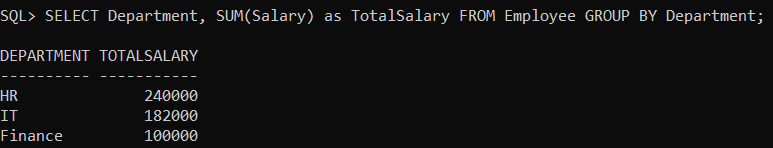##### GROUP BY Clause with MAX and MIN Function in Oracle

Now let us see an example of using GROUP BY Clause with MAX and MIN aggregate function in Oracle. The MAX aggregate function in Oracle is used to return the max value whereas the MIN aggregate function is used to return the Min value.

###### Example: Find the Highest and Lowest salary in Each Department in the Employee table

Here first we need to group the employees by department and then we need to execute the MAX and MIN aggregate functions on each group. And to the MAX and MIN aggregate function, we need to pass the Salary column as shown in the below SQL Query.

```SELECT Department, MAX(SALARY) as MaxSalary, MIN(SALARY) as MinSalary
FROM Employee
GROUP BY Department;```

Once you execute the above SQL query, you will get the following output which is showing the department name along with the highest and lowest salary of that department.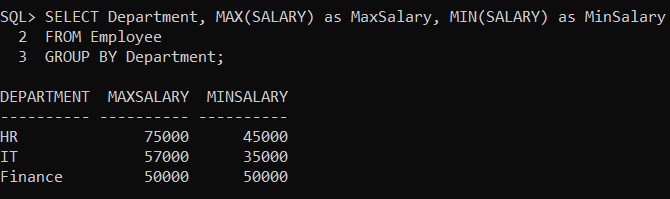##### GROUP By Clause with Multiple Columns in Oracle:

It is also possible to use Group by clause on Multiple Columns in Oracle. In that case, first, data in the table is divided based on the first column of the group by clause and then each group is subdivided based on the second column of the group by clause and then the aggregate function is applied to each inner group to get the desired result.

###### Example: Find the number of employees working in each Gender per Department

So, what our requirement is, first we need to group the employee by the department and then we need to group the employee by Gender present in each department. The following SQL query will exactly do the same.

```SELECT Department, Gender, COUNT(*) AS EmployeeCount
FROM Employee
GROUP BY Department, Gender
ORDER BY Department;```

When you execute the above query, you will get the following output.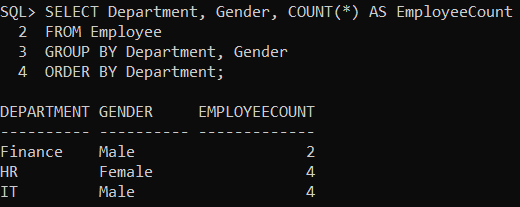###### Example: Find the total salaries and the total number of employees by City, and by gender

Here, we need to group by City first and then by Gender. Then we need to apply the SUM Aggregate function on the Salary column to calculate the total sum and the Count Aggregate function on the Id column to find the total number of employees.

```SELECT City, Gender, SUM(Salary) as TotalSalary, COUNT(ID) as TotalEmployees
FROM Employee
GROUP BY CITY, Gender;```

When you execute the above query, you will get the following output.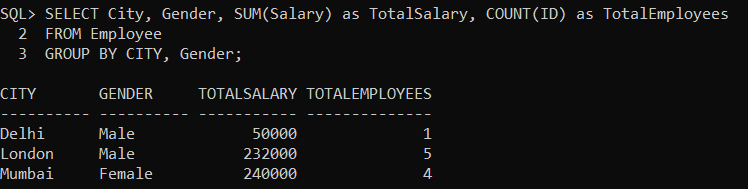##### GROUP BY Clause with AVG Function in Oracle

Now let us see an example of using Group by Clause with AVG aggregate function in Oracle. The AVG aggregate function in Oracle is going to return the average value of the column on which it is operated.

###### Example: Find the average salary of each department

Here first we need to group the employees by department and then we need to execute the AVG Aggregate function on each group. And to the AVG Aggregate function we need to pass the Salary column as shown in the below SQL Query.

```SELECT Department, AVG(Salary) as AverageSalary
FROM Employee
GROUP BY Department;```

Once you execute the above query, you will get the following output which is showing the department name along with the average salary of that department.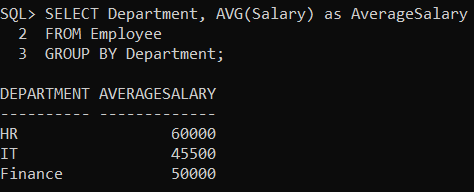Note: When the aggregate function is applied to a group, it returns only a single value but each group can return a value. Use Group By clause only on a column that contains duplicate values, never applies it on unique columns.

In the next article, I am going to discuss the HAVING Clause in Oracle with Examples. Here, in this article, I try to explain the GROUP BY Clause in Oracle with Examples and I hope you enjoy this GROUP BY Clause in Oracle with Examples article.